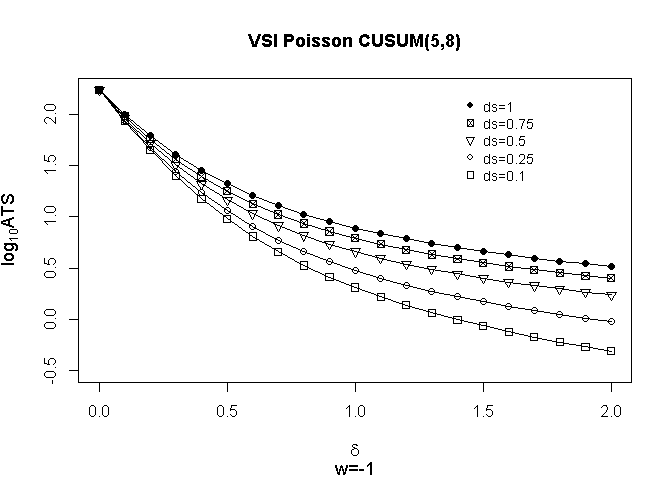# Tools for Attribute VSI CUSUM Charts

#### 2016-12-29

The package attrCUSUM has been developed to aid in the statistical design of attribute VSI CUSUM chart. It currently provides tools for monitoring of mean increase when the data are (zero inflated) Poisson/binomial distribution. These tools can be easily applied to other count processes and more complicated CUSUM control chart. We leave these issues as our perpetual work.

This vignette briefly illustrates some examples for usage of functions in the package.

## CUSUM statistic

Note that the considered CUSUM statistic for monitoring of mean increase is defined as follows:

$${C}_{0} = {c}_{0},$$

$${C}_{t} = \max \left( 0, {C}_{t-1}\right) + X_{t} - k, ~~ t \in \mathbf{N}$$,

wherein $$X_{t}$$ is a count process of interest, $$k$$ is the reference value and $$C_{0}$$ is the initial state.

## Getting ANSSs, ATSs and other informations

First of all, the package should be attached.

library(attrCUSUM)

Suppose that $$X_{i}$$ is distributed Poisson with mean $$\lambda$$. The function getAve_poisson provides useful information on design of upper one-sided CUSUM control chart when the data are Poisson.

Assume that we are interested in the average number of sample to signal (ANSS) an out-of-condition for CUSUM(k=5,h=8) with initial state 0 when the data are Poisson with mean 4 where k and h are the reference value and the (upper) control limit of CUSUM control chart respectively. Then the ANSS of CUSUM(k=5, h=8) chart can be obtained as follows:

lambda <-  4  # mean of Poisson distribution
k <- 5  # reference value
h <- 8  # control limit
c.zero <- 0  # initial state
res <- getAve_pois(lambda = lambda, refv = k, contl = h, c.zero = c.zero)
res$ANSS ##  171.7792 Next, Assume that we want to get the corresponding longer sampling time when the predetermined shorter sampling interval is 0.1 and the warning limit is -1. The results can be obtained as follows: lambda <- 4 # mean of Poisson distribution k <- 5 # reference value h <- 8 # control limit c.zero <- 0 # initial state warnl <- -1 # warning limit ds <- 0.1 # shorter sampling interval res1 <- getAve_pois(lambda = lambda, refv = k, contl = h, c.zero = c.zero, warnl = warnl, ds = ds) dl <- res1$dl  # longer sampling interval
dl
##  2.910912
res2 <- getAve_pois(lambda = lambda, refv = k, contl = h,
c.zero = c.zero, warnl = warnl, ds = ds, dl = dl)
res2$ANSS ##  171.7792 res2$ATS
##  171.7792

The following is an example of ATS profiles. Evaluate example(package = "attrCUSUM", "getAve_pois") for more details. In plot, $$\delta$$ denotes mean shift in units of standard deviation.Note that the function getAve_pois provides other useful information related to Markov chain approach. Refer to help(getAve) for more details.

names(res2)
##   "endpoints"       "numsubI"         "lensubI"
##   "Q"               "I_minus_Q"       "I_minus_Q_inv"
##   "I_minus_Q_inv_1" "initpr"          "ds"
##  "dl"              "di"              "psi.s"
##  "psi.l"           "refv.act"        "contl.act"
##  "warnl.act"       "c.zero.act"      "ANSS"
##  "ATS"

## Getting suitable control limit

As was before, Assume that $$X_{i}$$ is distributed Poisson with mean $$\lambda$$, also we want to get suitable control limit when the data are Poisson with mean 4 for that the reference value is 5, the initial state is 0 and the desired ANSS is near 200. Then, the suitable control limit can be obtained as follows:

lambda <-  4  # mean of Poisson distribution
k <- 5  # reference value
c.zero <- 0  # initial state
anss.target <- 200L  # desired anss
getContl_pois(lambda = lambda, anss.target = anss.target,
refv = k, c.zero = c.zero)
## $refv.act ##  5 ## ##$c.zero.act
##  0
##
## $sol1 ## contl1 ANSS1 ## 8.0000 171.7792 ## ##$sol2
##   contl2    ANSS2
##   9.0000 270.0112

## Other count process

Although we illustrated only the case that the data are Poisson, the package attrCUSUM also provides other count processes.

• For (zero-inflated) binomial VSI CUSUM chart, refer to help(getAve_binomial) and help(getContl_binomial).
• For (zero-inflated) Poisson VSI CUSUM chart, refer to help(getAve_Poisson) and help(getContl_Poisson).
• For user-specified VSI CUSUM chart, refer to help(getAve) and help(getContl).

• ‘attrCUSUM’ provides functions for density, distribution, quantile and random generation for the zero inflated Poisson distribution. Refer to help(zipois)
• ‘attrCUSUM’ provides functions for density, distribution, quantile and random generation for the zero inflated binomial distribution. Refer to help(zibinom)